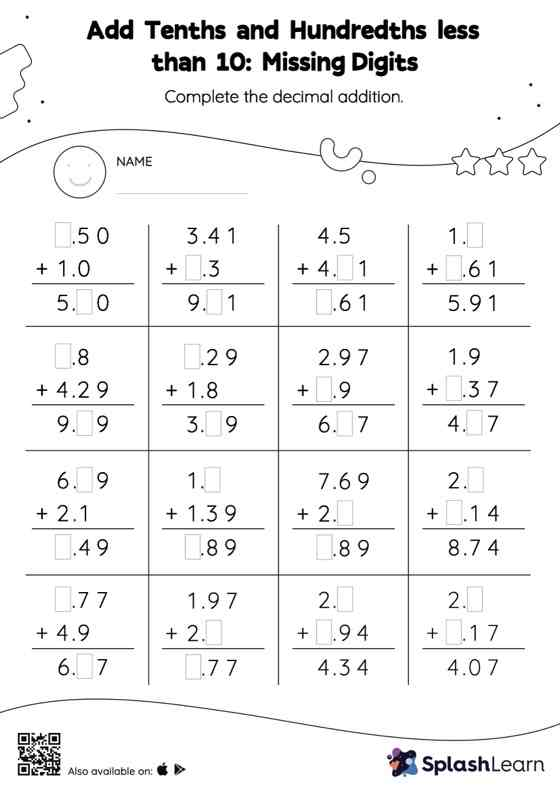# Add Tenths and Hundredths less than 10: Missing Digits Worksheet

Home > Add Tenths and Hundredths less than 10: Missing DigitsStudents align the decimal points and use zero as space holders to add decimals. Then they use the relationship between addition and subtraction to find the missing number in add tenths and hundredths less than 10 worksheet. This worksheet is about practicing the column method in which numbers are written one on top of another. In this method, students use their place value understanding to solve the problems.